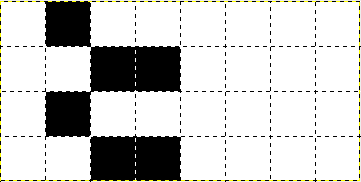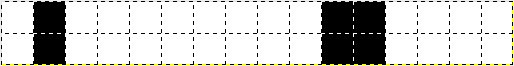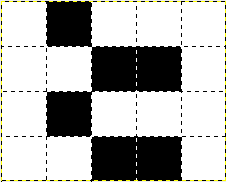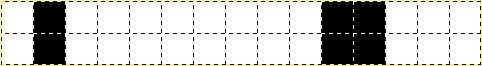# PBM P4 image file format

## What is PBM P4?

PBM P4 is a hybrid monochrome file format, a mixture of ASCII and binary data.
It can be opened by several imaging software (eg. Gimp).

## PBM P4 structure

Source of example.pbm :

```P4
# CREATOR: bitmap2pbm Version 1.0.0
8 4 @0@0```

The file starts with the ASCII section and ends with the binary section.

### ASCII section

“P4” is the magic word which identifies the file format
The line starts with “#” is a comment (optional).
“8 4” is the dimension of the binary data
The ASCII section ends with exactly one whitespace after dimension

A character is a whitespace if isspace() returns true for it.
A comment line must end with CR of LF.
Any number of whitespaces could appear between the tokens.

### Binary section

Contains the image data, where every bit corresponds to one pixel.
The example file consists of “@0@0” which is 4 bytes => 32 bits total.
Character code of “@” is 64, which is 01000000 in binary.
Character code of “0” is 48 which is 00110000 in binary.

“8 4” dimension means 4 lines of 8 bit binary data.“16 2” dimension means 2 lines of 16 bit binary data.BUT!

“5 4” dimension means 4 lines of 8 bit binary data, where only the most significant 5 bits are used.“15 2” dimension means 2 lines of 16 bit binary data, where only the most significant 15 bits are used.With lines there are no problems, they can be anything > 0.